# OECD Purchasing power parities (PPP) data analysis 3 - relationship with GDP data and PPP. Some countries have positive correlation and some have negative.Photo by Quino Al on Unsplash

This post is following of the above post.
I have GDP data file like below, which I downloaded OECD web site.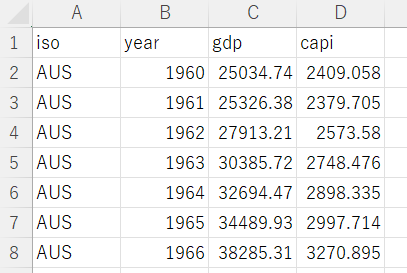I am going to merge this data to previous ppp data.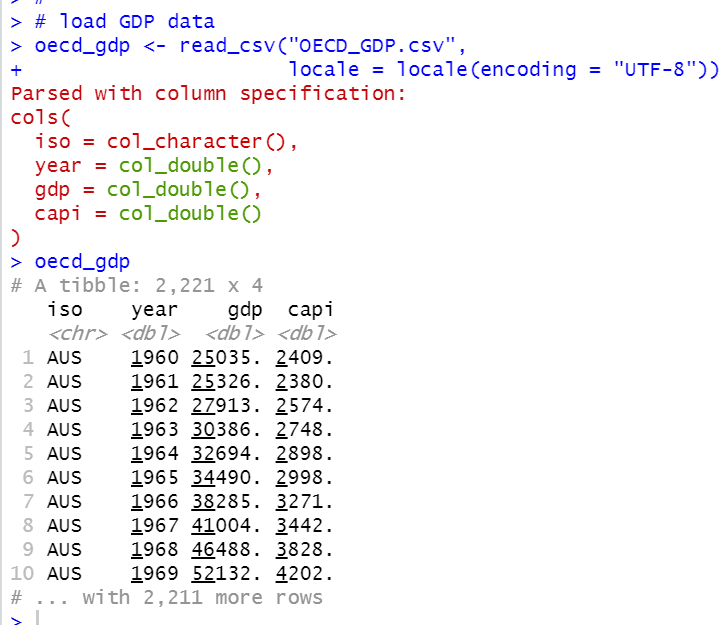Then, I use inner_join() function to mergr previous dataframe;df and this GDP dataframe;oecd_gdp.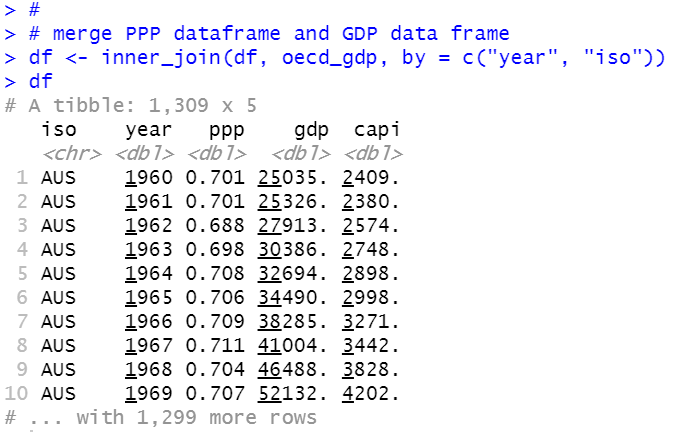gdp is real GDP and capi is per capita GDP.

Let's see ppp and gpd graph.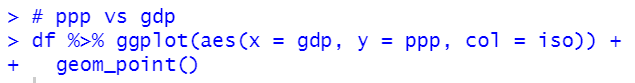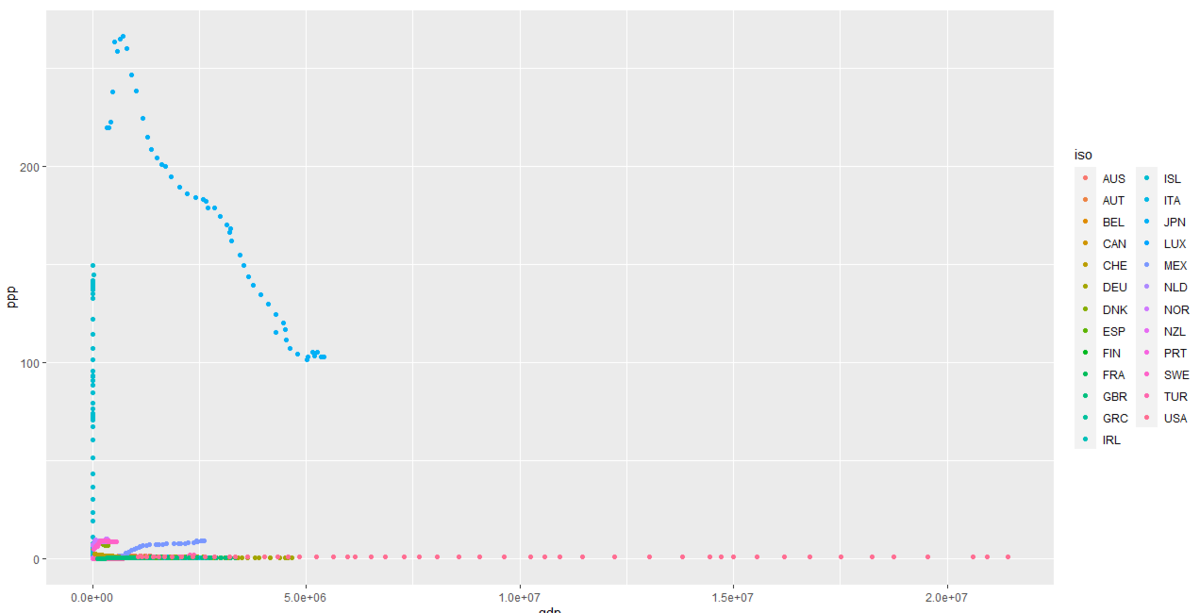Since GDP and PPP have wide range data, above graph is not good to see.

I change ppp to log(ppp) and gdp to log(gdp).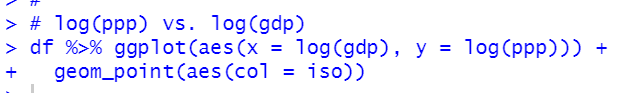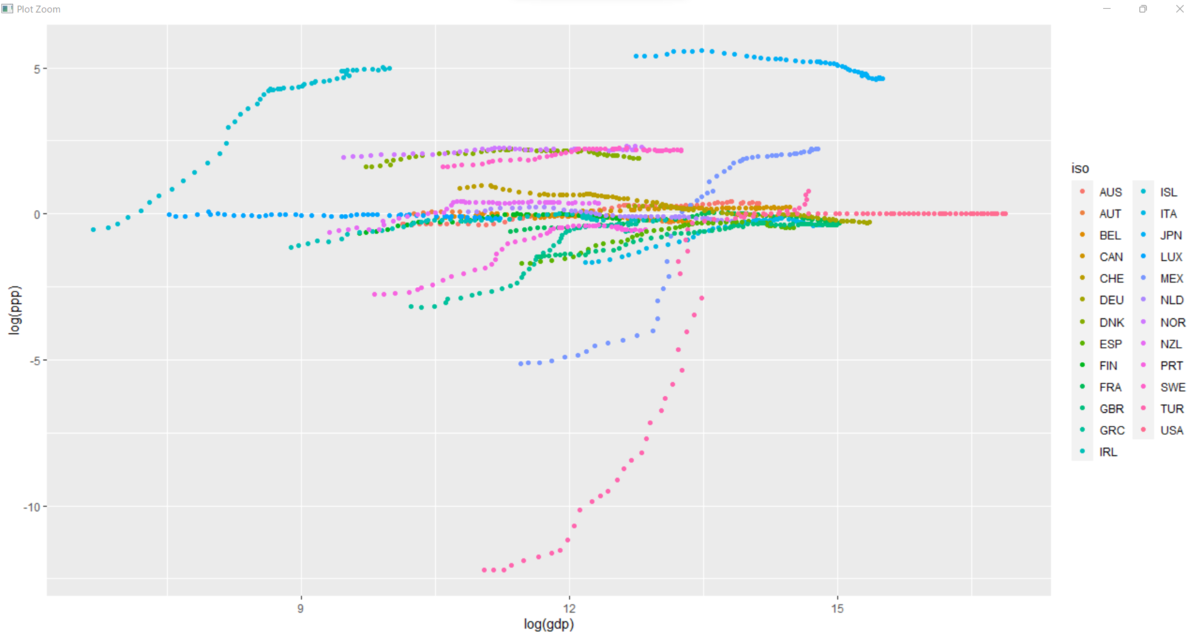I see many countries have positive correlation between log(gdp) and log(ppp).

Now, let's see ppp and capi: per capita GDP.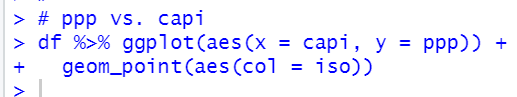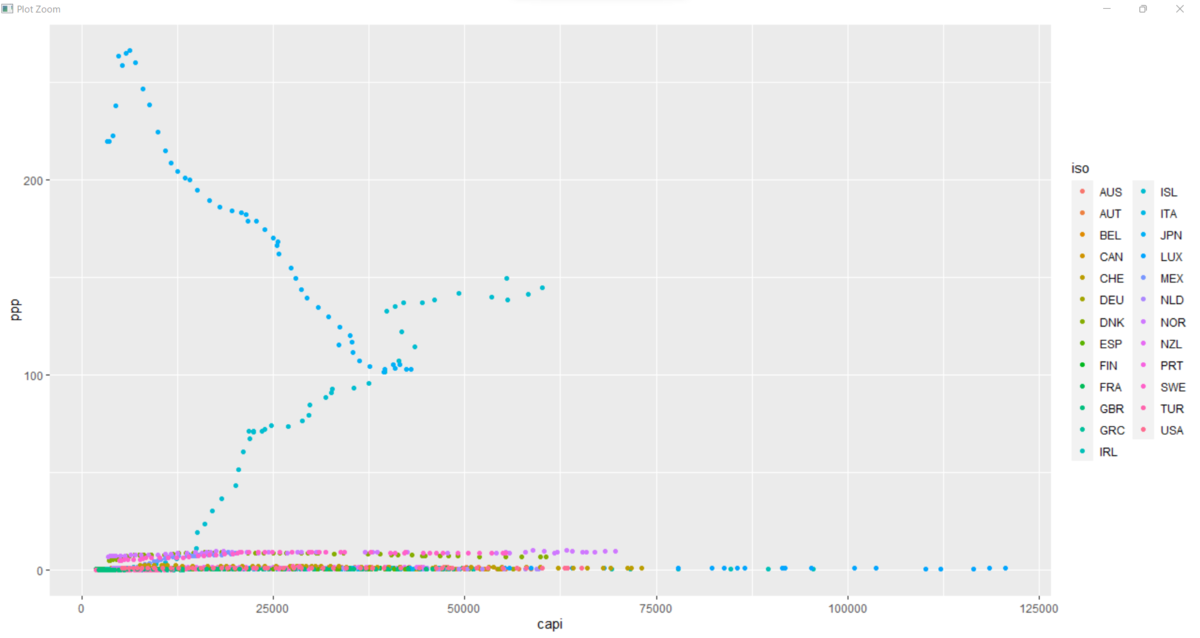Again, let's convert ppp to log(ppp) and capi to log(capi).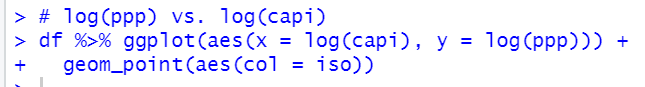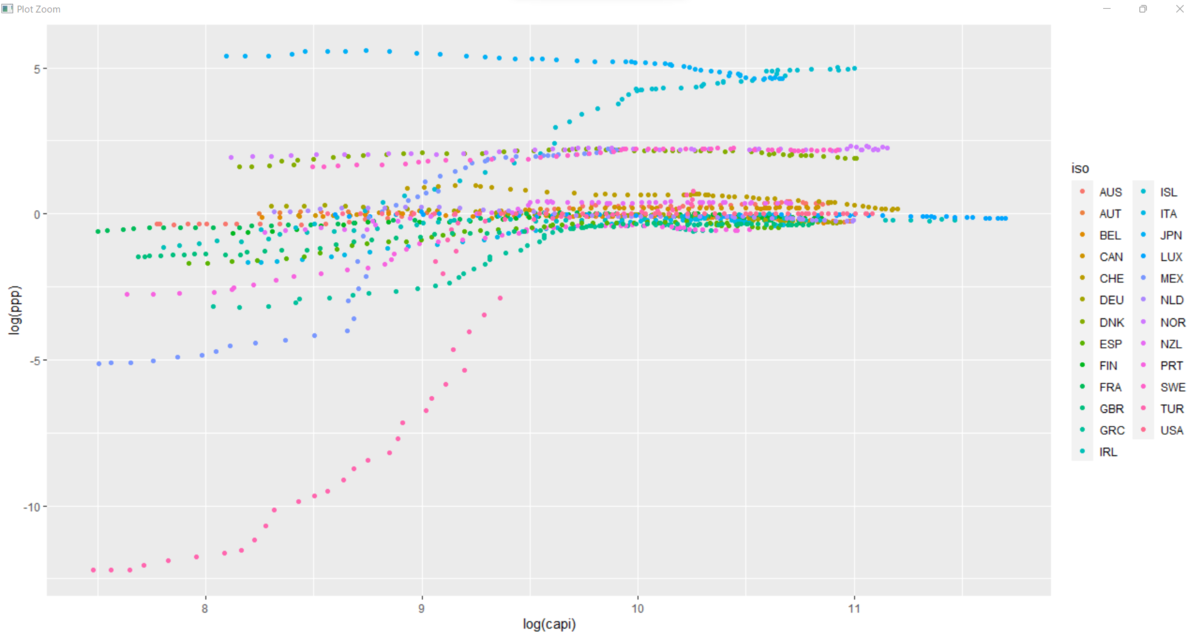At this point, I realize it is better to see log(ppp), log(gdp) and log(capi) than ppp, gdp and capi in order to analyze them, I am going to make a new vatiables.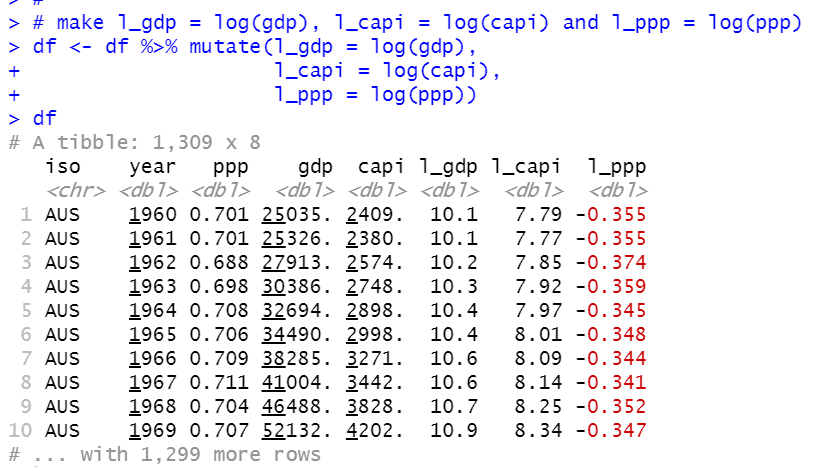l_ppp is log(ppp), l_gdp is log(gdp) and l_capi is log(capi).

Let's see correlation between l_ppp and l_gdp by year.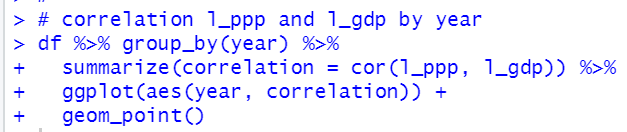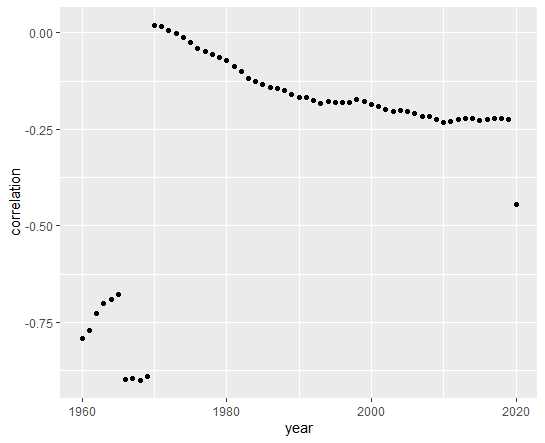We see 1960 ~ 1969 and 2020 are different characteristics than other years. Correlation between log(gdp) and log(ppp) seems negative correlation.

Let's see log(ppp) and log(capi) by year.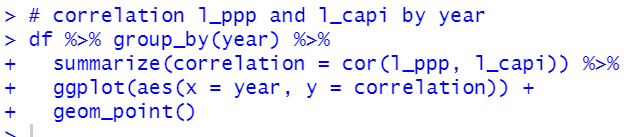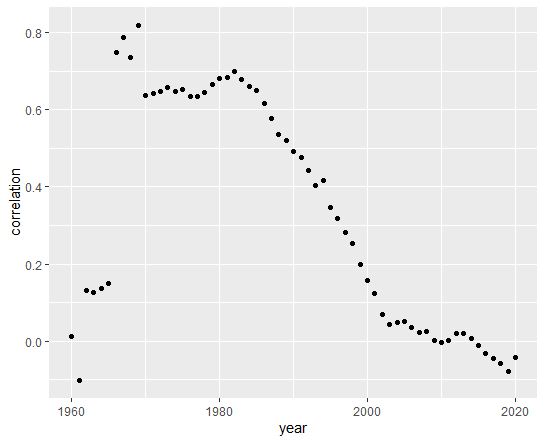We see 1960 ~ 1969 and 2020 are silightly different characteristics. Correlation between log(ppp) and log(capi) seems positive correlation.

I make df subset for excluding 1960 ~ 1969 and 2020.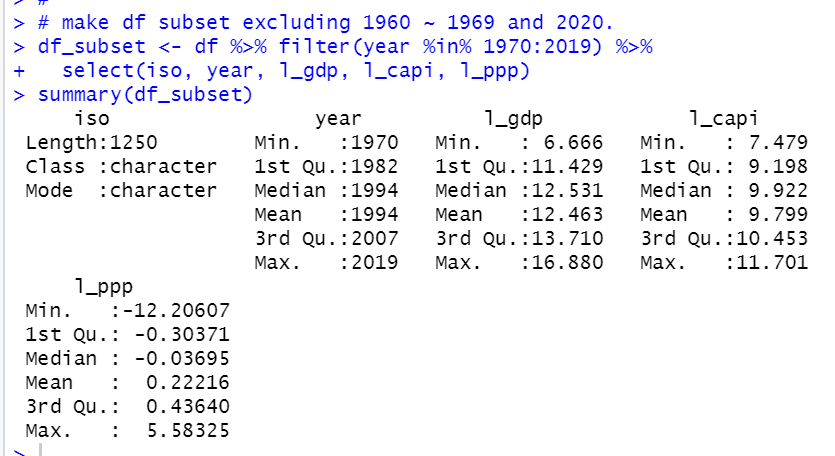Using this df_subset, let's see l_ppp and l_gdp correlation by country.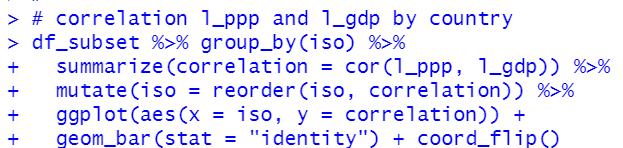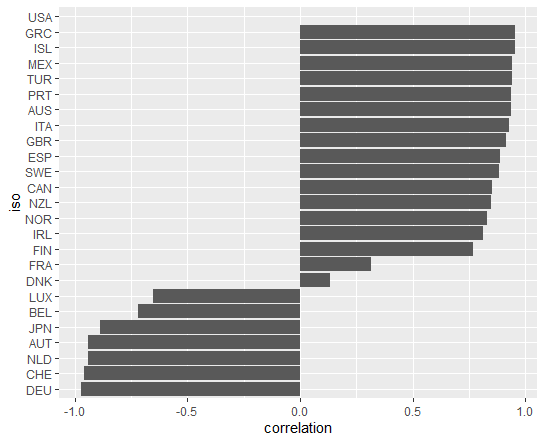I see USA is excluded because USA has no variation for l_ppp. Some countries have positive correlation and some has negative correlation.

I will delete USA from df_subset and let's see l_ppp and l_capi by country.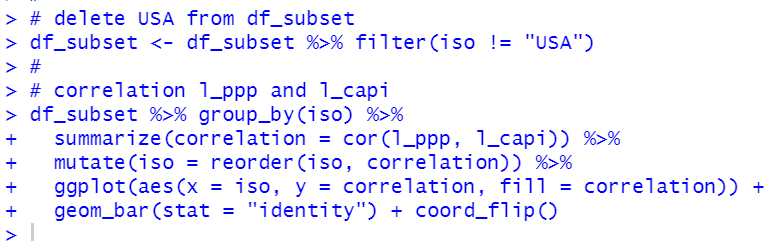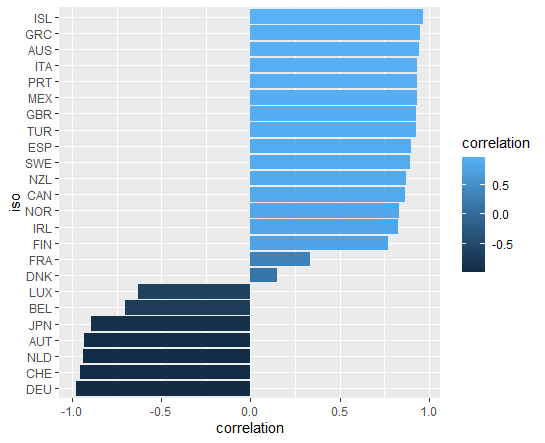Like l_ppp and l_gdp, some contries have positive correlation between l_ppp and l_capi and some have negative correlation.

That's it. Thank you!

The next post is